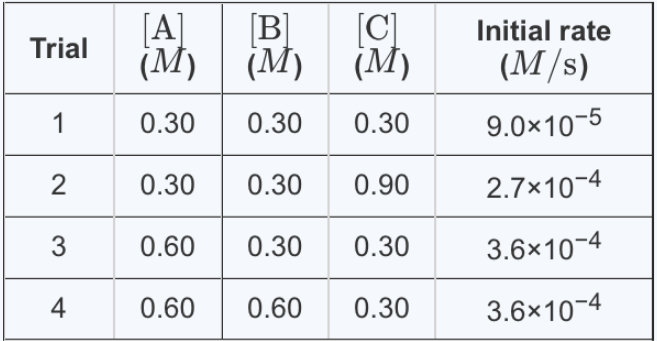# Problem: For the reaction A +B+ C → D + E, the initial reaction rate was measured for various initial concentrations of reactants. The following data were collected:What is the value of the rate constant k for this reaction? Express your answer to two significant figures and include the appropriate units.

###### FREE Expert Solution
95% (232 ratings)
###### Problem Details

For the reaction A +B+ C → D + E, the initial reaction rate was measured for various initial concentrations of reactants. The following data were collected:

What is the value of the rate constant k for this reaction? Express your answer to two significant figures and include the appropriate units.Frequently Asked Questions

What scientific concept do you need to know in order to solve this problem?

Our tutors have indicated that to solve this problem you will need to apply the Rate Law concept. You can view video lessons to learn Rate Law. Or if you need more Rate Law practice, you can also practice Rate Law practice problems.

What is the difficulty of this problem?

Our tutors rated the difficulty ofFor the reaction A +B+ C → D + E, the initial reaction rate ...as high difficulty.

How long does this problem take to solve?

Our expert Chemistry tutor, Dasha took 12 minutes and 16 seconds to solve this problem. You can follow their steps in the video explanation above.

What professor is this problem relevant for?

Based on our data, we think this problem is relevant for Professor Clizbe's class at UB.